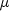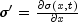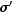JMSLTM Numerical Library 7.2.0
com.imsl.math

## Interface FeynmanKac.PdeCoefficients

• Enclosing class:
FeynmanKac

`public static interface FeynmanKac.PdeCoefficients`
Public interface for user supplied PDE coefficients in the Feynman-Kac PDE.
• ### Method Summary

Methods
Modifier and Type Method and Description
`double` ```kappa(double x, double t)```
Returns the value of thecoefficient at the given point.
`double` ```mu(double x, double t)```
Returns the value of thecoefficient at the given point.
`double` ```sigma(double x, double t)```
Returns the value of thecoefficient at the given point.
`double` ```sigmaPrime(double x, double t)```
Returns the value ofat the given point.
• ### Method Detail

• #### kappa

```double kappa(double x,
double t)```
Returns the value of thecoefficient at the given point.

Time dependency ofcan be controlled via method `setTimeDependence`. Use of this method will usually yield a more efficient algorithm because some finite element matrices do not have to be reassembled for each `t` value.

Parameters:
`x` - a `double`, the point in space at whichis to be evaluated.
`t` - a `double`, the time point at whichis to be evaluated.
Returns:
a `double`, the value ofat `(x,t)`.
• #### mu

```double mu(double x,
double t)```
Returns the value of thecoefficient at the given point.

Time dependency ofcan be controlled via method `setTimeDependence`. Use of this method will usually yield a more efficient algorithm because some finite element matrices do not have to be reassembled for each `t` value.

Parameters:
`x` - a `double`, the point in space at whichis to be evaluated.
`t` - a `double`, the time point at whichis to be evaluated.
Returns:
a `double`, the value ofat `(x,t)`.
• #### sigma

```double sigma(double x,
double t)```
Returns the value of thecoefficient at the given point.

Time dependency ofcan be controlled via method `setTimeDependence`. Use of this method will usually yield a more efficient algorithm because some finite element matrices do not have to be reassembled for each `t` value.

Parameters:
`x` - a `double`, the point in space at whichis to be evaluated.
`t` - a `double`, the time point at whichis to be evaluated.
Returns:
a `double`, the value ofat `(x,t)`.
• #### sigmaPrime

```double sigmaPrime(double x,
double t)```
Returns the value ofat the given point.

Time dependency ofcan be controlled via method `setTimeDependence`. Use of this method will usually yield a more efficient algorithm because some finite element matrices do not have to be reassembled for each `t` value.

Parameters:
`x` - a `double`, the point in space at whichis to be evaluated.
`t` - a `double`, the time point at whichis to be evaluated.
Returns:
a `double`, the value ofat `(x,t)`.
JMSLTM Numerical Library 7.2.0